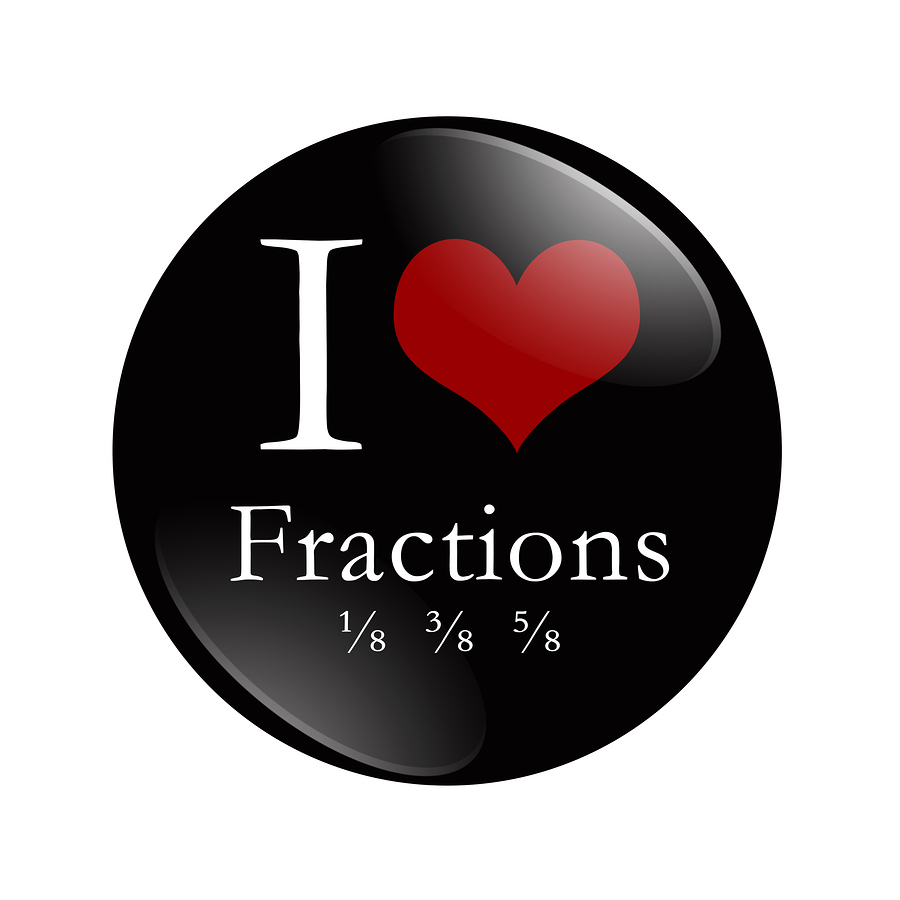By |October 6th, 2015|CCSS, Ed Talks, Math, STEM, Teaching & Learning|After spending time with secondary math teachers, I have come to believe that students’ success in Algebra 1 is directly related to their ability to work confidently with fractions. The Common Core State Standards (CCSS) calls out fractions as a “top-level organizer.” Numbers and Operations with Fractions have been granted their own domain and are grounded in conceptual understanding with a focus on equivalency and multiple representations.

Number sense around fractions begins to surface in Kindergarten, in the Geometry domain, where standards ask students to compose a rectangle out of two equivalent triangles. Primary students make observations around measurement in context using words like “half way there” and “one third of the cookie” to become comfortable with fractions. Second graders represent whole numbers on the number line to show measurement thus becoming familiar with the number line as a tool to use later with fractions. Second graders also partition circles and rectangles to talk about equal shares such as, 3/4 of a circle or 2/3 a rectangle.

In third grade, students begin to develop the concept of the unit fraction as the building block for all fractions: those less than one, those equal to one and those greater than one. They compare unit fractions and specify the related whole; they are called to explain, “ equal parts” in context of the whole. Third graders use visual fraction models focused on equal numerators and denominators to compare fractions.

As students enter fourth grade, students create models to demonstrate fraction equivalence. They compose and decompose fractions through addition of unit fractions and multiplication of unit fractions by a whole number. Fourth graders also look at models of equivalent fractions and the how multiplying the numerator and denominator by the same value affects the partitioning of their model. In grade four, students also study and compare at decimal fractions. Application problems with fractions continue and involve addition, subtraction of fractions with common denominators. The Measurement and Data standards ask students use line plots with units of 1/2, 1/4, and 1/8. The support of fraction sense-making for students in supporting domains, speaks to the coherence of the CCSS.

As students move into fifth grade, they use their knowledge of equivalency and their models for adding and subtraction fractions to add and subtract fractions with unlike denominators. They estimate sums and differences of fractions and build models of multiplication and division of fractions. Like in previous grades, students are asked to apply a context to a “naked number” fraction equation involving the four operations. Fifth graders explore the inverse relationship between multiplication and division of fractions, thus extending the inverse relationship found when multiplying and dividing whole numbers and preparing for important work in Algebra.

Deep analyses of fractions continues as students move into sixth and seventh grade and realize that fractions, decimals and percents can all be compared to a part to whole ratio. The CCSS in the middle grades focus on Ratio and Proportional Relationships and Expressions and Equations which draw on students understanding of fractions as numbers and ratios. They are asked to consider ratios that represent proportional relationships and look at the idea of scaling. When students work with congruence and similarity in Geometry or rational exponents in Algebra, they again draw on their experiences with fractions.

As teachers of mathematics, we must present our students with multiple exposures to fractions in a context that is appropriate and engaging for their grade level. We must ask our student to precisely explain how they are seeing the fraction problem and why they chose a particular strategy or model to solve the problem. And, at each grade level, students must be asked to deepen their overall mathematical sense-making through their work with fractions.